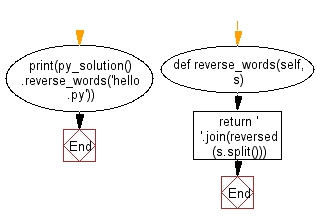﻿ Python: Reverse a string word by word - w3resource# Python: Reverse a string word by word

## Python Class: Exercise-8 with Solution

Write a Python class to reverse a string word by word.

Sample Solution:

Python Code:

``````class py_solution:
def reverse_words(self, s):
return ' '.join(reversed(s.split()))

print(py_solution().reverse_words('hello .py'))
```
```

Sample Output:

```.py hello
```

Pictorial Presentation:Flowchart:## Visualize Python code execution:

The following tool visualize what the computer is doing step-by-step as it executes the said program:

Python Code Editor:

What is the difficulty level of this exercise?

Test your Programming skills with w3resource's quiz.

﻿

## Python: Tips of the Day

The Zip() Function:

```>>> students = ('John', 'Mary', 'Mike')
>>> ages = (15, 17, 16)
>>> scores = (90, 88, 82, 17, 14)
>>> for student, age, score in zip(students, ages, scores):
...     print(f'{student}, age: {age}, score: {score}')
...
John, age: 15, score: 90
Mary, age: 17, score: 88
Mike, age: 16, score: 82
>>> zipped = zip(students, ages, scores)
>>> a, b, c = zip(*zipped)
>>> print(b)
(15, 17, 16)
```